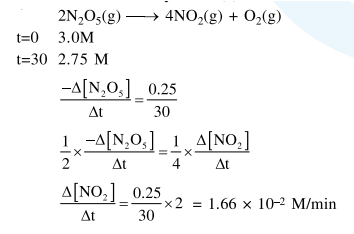# NO2 required for a reaction is produced by

Question:

$\mathrm{NO}_{2}$ required for a reaction is produced by the decomposition of $\mathrm{N}_{2} \mathrm{O}_{5}$ in $\mathrm{CCl}_{4}$ as per the equation

$2 \mathrm{~N}_{2} \mathrm{O}_{5}(\mathrm{~g}) \rightarrow 4 \mathrm{NO}_{2}(\mathrm{~g})+\mathrm{O}_{2}(\mathrm{~g})$

The initial concentration of $\mathrm{N}_{2} \mathrm{O}_{5}$ is $3.00 \mathrm{~mol} \mathrm{~L}^{-1}$ and it is $2.75 \mathrm{~mol} \mathrm{~L}^{-1}$ after 30 minutes. The rate of formation of $\mathrm{NO}_{2}$ is :

1. $2.083 \times 10^{-3} \mathrm{~mol} \mathrm{~L}^{-1} \mathrm{~min}^{-1}$

2. $4.167 \times 10^{-3} \mathrm{~mol} \mathrm{~L}^{-1} \mathrm{~min}^{-1}$

3. $8.333 \times 10^{-3} \mathrm{~mol} \mathrm{~L}^{-1} \mathrm{~min}^{-1}$

4. $1.667 \times 10^{-2} \mathrm{~mol} \mathrm{~L}^{-1} \mathrm{~min}^{-1}$

Correct Option: , 4

Solution: